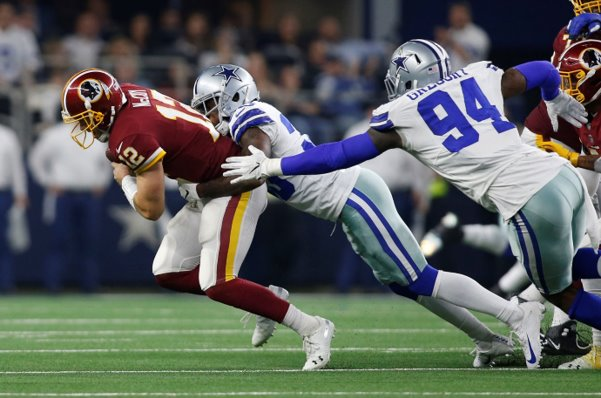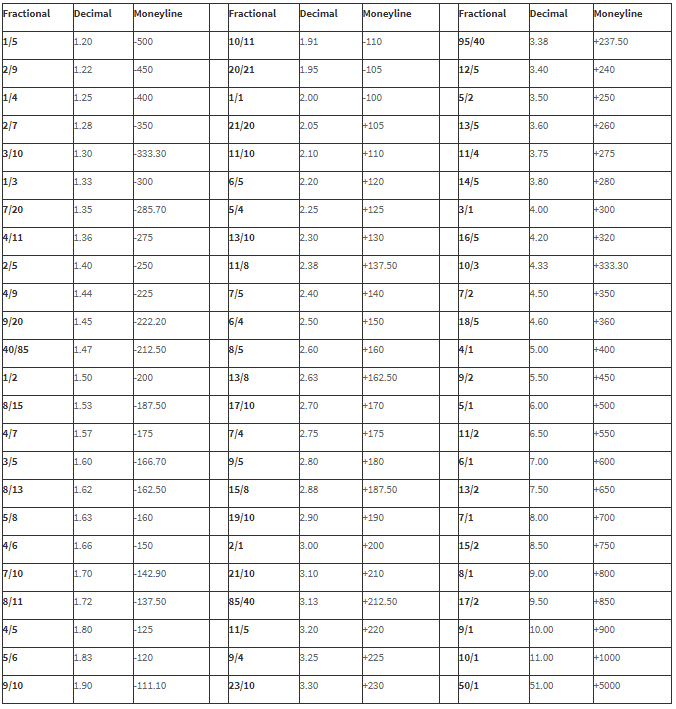# Understanding Decimal Odds

There is a lot of jargon used in sports betting and it can be quite confusing, especially for a novice, but once you get to grips with the basic terminology, your betting experience will be enhanced. The first thing that any punter needs to do is understand how the odds work and what they mean.

Many betting websites - including the Betfair Exchange - now use decimal odds as standard, so let’s take a closer look at this popular format.## The purpose of sports betting odds

Odds are a numerical way of describing the probability of an outcome occurring. The odds are calculated by bookmakers like Betfair and Paddy Power in a way that allows them a small profit margin. For example, on a 50/50 coin toss, a bookmaker might offer odds of 49/50. If they get an equal amount of money bet on heads and tails, they would be guaranteed a small profit whatever the outcome.

[ TOP 3 SIGN UP OFFERS WIDGET ]

## What are decimal odds?

Decimal odds are a way of displaying odds in an easy to understand way.

In the above example, where the bookmaker offers odds of 49/50 on a coin toss, the odds are shown as a fraction. If we showed the same odds in decimal format, they would look like this:

• Decimal odds: 1.98

So, if a punter placed a stake of £1 on this bet and it was successful, they would receive £1.98 back, achieving a profit of 98p.In decimal odds, odds-on selections will be expressed as values between 1.0 and 2.0

Many websites use the decimal format as standard when displaying the prices of sporting events. In fact, decimal odds are commonly used all over Europe. They are popular because they are so easy to understand, displaying the total return from a 1 unit stake.

As the unit stake is always included, decimal odds always have a value greater than 1.

Here is another example of how simple it is to read decimal odds:

• Odds: 6.0
• Stake: £1
• Winnings: £5
• Total return: £6

## Decimal vs. fractional odds

Punters in the UK and Ireland might not be as familiar with decimal odds. In those regions, it is more common to see fractional odds such as 5/1 or 9/2. Fractional odds basically describe the winnings from a theoretical stake.Fractional odds example:

• Odds: 5/1
• Stake: £1
• Winnings: £5
• Total return: £6

Where the odds are 50/50, the odds are described as Evens rather than 1/1.

## The advantage of decimal odds

Fractional odds can sometimes be confusing as the calculation can produce very large numbers. For example, where the decimal odds are 1.46, the fractional equivalent would be 23/50. In this case, it is much easier to calculate the return from a £1 stake by using the decimal odds format.

In addition, fractional odds can be confusing when the probability is very high. For example, when decimal odds fall below 2, the fractional equivalent suddenly takes a different appearance as the second number becomes larger than the first number. Therefore, it is possible to have fractional odds of 2/1 and 1/2 and this can be a little confusing for novice punters.

## Decimal vs. moneyline odds

Moneyline odds (also known as US or American odds) are used by bookmakers in America. The odds are presented in either a negative or positive format depending on the probability.Moneyline odds are used by bookies in America

Positive moneyline odds show how much would be won from a \$100 stake. In a sporting event involving two teams, the underdog always has a positive moneyline.

Positive moneyline example:

• Odds: +500
• Stake: \$100
• Winnings: \$500
• Total return: \$600

Negative moneyline odds show how much would need to be staked to win \$100. In a sporting event involving two teams, the favourite always has a negative moneyline.

Example

• Odds: -500
• Stake: \$500
• Winnings: \$100
• Total return: \$600

If there is no clear favourite and the odds are 50/50, the moneyline is displayed as +100. Using the positive rule, a \$100 bet would then return a \$100 profit.

Converting moneyline odds to decimal or fractional odds is not always easy. However, the tools below can be used as a handy guide.## Why is understanding odds important?

Understanding how to read odds is a vital part of sports betting. If you do not know how to read the odds, you will never know if you are getting good value or what your potential returns might be.

In theory, decimal odds are the easiest to understand and many punters have switched to using decimal odds after years of using the fractional format. Decimal odds can be read at a glance and do not require any tricky calculations.

Most betting websites - including Betfair and Paddy Power- have a settings menu where you can change the way that odds are displayed. This means you can choose the option that suits you best. But even if you prefer to use fractional odds, it still makes sense to learn how to read decimal odds as they are becoming more widely used across the internet.

Check out the latest horse racing odds for today's races over on the TImeform horse racing site or get odds for today's football matches at Infogol.net.

[ TOP 3 BETTING OFFERS WIDGET ]